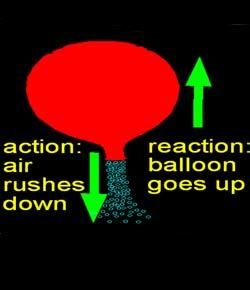# Chapter 10 Quiz Motion

35 Questions | Attempts: 3725
ShareSettingsThis is the chapter 10 test.   You may not use any items except for a calculator and a sheet of paper for working problems.

• 1.
Speed in a specific direction is
• A.

Motion

• B.

Position

• C.

Locatoin

• D.

Velocity

• E.

Accleration

• 2.
A change of position.
• A.

Motion

• B.

Location

• C.

Speed

• D.

Velocity

• E.

Acceleration

• 3.
A change in position over time is....
• A.

Location

• B.

Position

• C.

Motion

• D.

Velocity

• E.

Acceleration

• 4.
An object's location is
• A.

Motion

• B.

Position

• C.

Speed

• D.

Velocity

• E.

Acceleration

• 5.
The location from where you measure the change in position is
• A.

Reference point

• B.

Position

• C.

Speed

• D.

Velocity

• E.

Acceleration

• 6.
The rate at which velocity changes over time is
• A.

Motion

• B.

Location

• C.

Speed

• D.

Velocity

• E.

Acceleration

• 7.
A quantity with both size and direction is
• A.

Speed

• B.

Velocity

• C.

Vector

• D.

Acceleration

• E.

Noodles

• 8.
A position describes an object's location compared to
• A.

Its motion

• B.

A reference point

• C.

Its speed

• D.

A vector

• 9.
Maria walked 2km in half an hour.  What was her average speed during her walk?
• A.

0.5 km/hr

• B.

1 km/hr

• C.

2 km/hr

• D.

4 km/hr

• E.

60 km/hr

• 10.
A vector is a quality that has
• A.

Speed

• B.

Acceleration

• C.

Size and direction

• D.

Position and distance

• 11.
Mary and Keisha run with the same constant speed but in opposite directions.  The girls have
• A.

The same position

• B.

Different accelerations

• C.

Different speeds

• D.

Different velocities

• 12.
A swimmer increases her speed as she approaches the end of the pool.  Her acceleration is
• A.

In the same direction as her motion

• B.

In the opposite direction of her motion

• C.

At right angles to her motion

• D.

Zero

• 13.
A cheetah can go from 0 m/s to 20 m/s in 2 s.  What is the cheetah's acceleration?
• A.

0.1 m/s2

• B.

2 m/s2

• C.

10 m/s2

• D.

20 m/s2

• E.

40 m/s2

• 14.
Jon walks for a few minutes, then runs for a few minutes.  During this time, his average speed is
• A.

The same as his final speed

• B.

Greater than his final speed

• C.

Less than his final speed

• D.

Constant velocity

• 15.
Suppose you are biking with a friend.  How would your friend describe your relative motion as he passes you?
• A.

You are moving backward

• B.

You are moving forward

• C.

You are not moving

• D.

You are speeding up

• 16.
A person sitting in a chair gets up and runs to the door at 10m/s in 2 seconds.  What is their acceleration?
• A.

10 m/s2

• B.

-10 m/s2

• C.

20 m/s2

• D.

-20 m/s2

• E.

5 m/s2

• 17.
An object traveling at 20 m/s2 experiences no acceleration.  Which one of these is true for the object.
• A.

The object gets faster

• B.

The object slows down

• C.

The object stops when it has no acceleration

• D.

The object keeps moving at the same speed

• 18.
An object speeds up to 100 m/s over the course of 10 seconds.  In order to calculate acceleration what other information do you need.
• A.

The objects initial speed

• B.

The objects final speed

• C.

The objects initial velocity

• D.

The objects final velocity

• E.

Nothing. You have all the necessary information

• 19.
A car goes from 20m/s to 30m/s in 10 seconds.  What is the acceleration?
• A.

600 m/s2

• B.

60 m/s2

• C.

30 m/s2

• D.

6 m/s2

• E.

1 m/s2

• 20.
A penguin going 10 m/s after 5 seconds is going 5 m/s.  What is the penguins acceleration
• A.

1 m/s2

• B.

-1 m/s2

• C.

10 m/s2

• D.

-10 m/s2

• E.

50 m/s2

• 21.
The space shuttle travels at 15,000 m/s in order to enter earth atmosphere it changes it's speed to 10,000 m/s over 100 seconds.  What is the space shuttles acceleration?
• A.

150 m/s2

• B.

-150 m/s2

• C.

50 m/s2

• D.

-50 m/s2

• E.

15,000 m/s

• 22.
Force has size and direction making it a
• A.

Velocity

• B.

Speed

• C.

Energy

• D.

Vector

• E.

Acceleration

• 23.
When you change direction you change
• A.

Speed and velocity

• B.

Vector and acceleration

• C.

Velocity and vector

• D.

Vector and speed

• E.

Veloctiy and acceleration

• 24.
A person traveling north 20 m/s would have what velocity south?
• A.

20 m/s

• B.

-20 m/s

• C.

20 m/s2

• D.

-20 m/s2

• E.

0 m/s

• 25.
A person changes their velocity south of - 10 m/s to 20 m/s in one second.  What is their acceleration?
• A.

-10 m/s2

• B.

0 m/s2

• C.

10 m/s2

• D.

20 m/s2

• E.

30 m/s2

## Related TopicsBack to top
×

Wait!
Here's an interesting quiz for you.